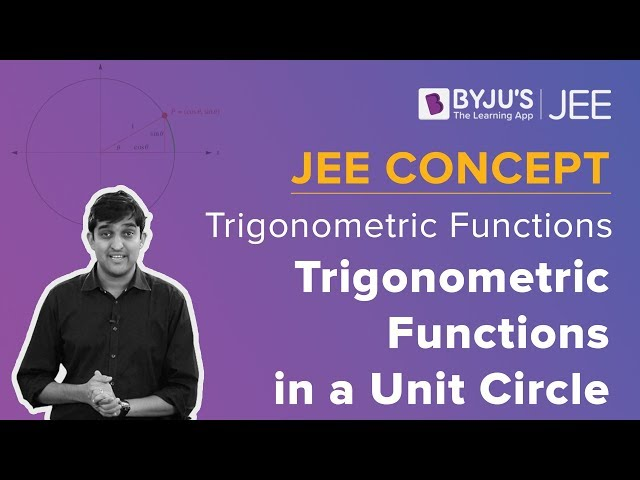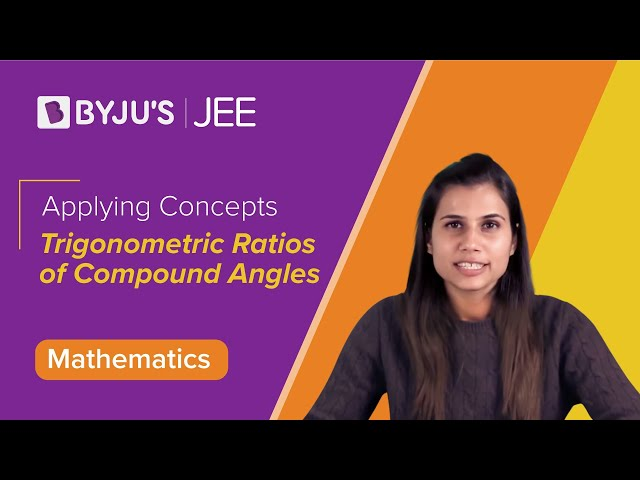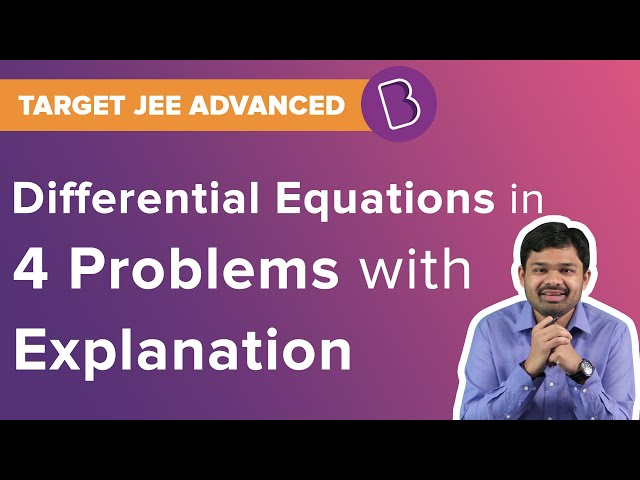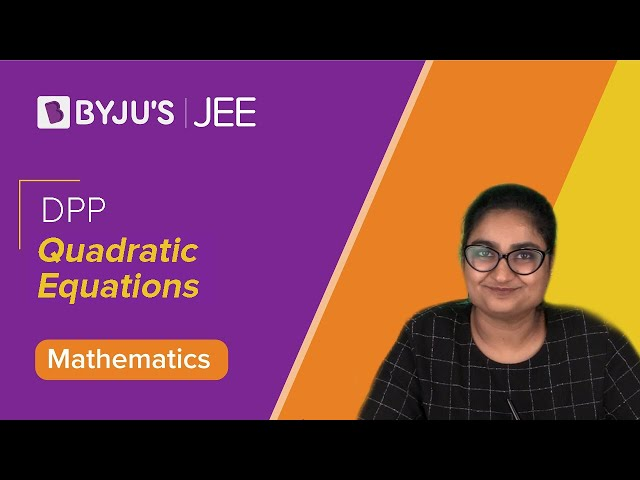Win up to 100% scholarship on Aakash BYJU'S JEE/NEET courses with ABNAT Win up to 100% scholarship on Aakash BYJU'S JEE/NEET courses with ABNAT

# JEE Main 2020 Maths Paper With Solutions Shift 2 Sept 6

JEE Main 2020 Maths paper September 6 Shift 2 solutions are available on this page. These are solved by experts at BYJU’S. Practising previous question papers is one of the best methods for JEE preparation. Students are recommended to revise and learn these solutions, so that they can easily crack the JEE Main exam. They can easily download the PDF format of these solutions for free.

## JEE Main 2020 Maths Paper With Solutions Shift 2 September 6

September 6 Shift 2 – Maths

1. If the normal at an end of a latus rectum of an ellipse passes through an extremity of the minor axis, then the eccentricity e of the ellipse satisfies:

1) e4+2e2-1 = 0
2) e2+2e-1 = 0
3) e4+e2-1 = 0
4) e2+e-1 = 0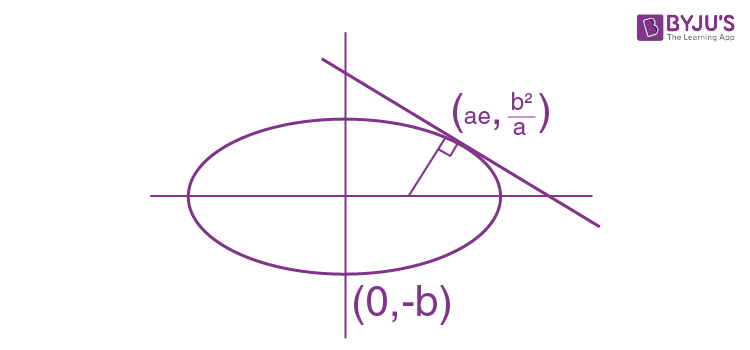Equation of normal at (ae, b2/a)

(a2x/ae)-b2y/(b2/a) = a2e2

(ax/e)-ay = a2e2

⇒ (x/e)-y = ae2

It passes through (0,-b)

-b = ae2 ⇒b2 = a2e4

a2 (1- e2 ) = a2e4

⇒ e4+e2-1 = 0

2. The set of all real values of λ for which the function f(x) = (1-cos2 x)(λ+sinx) , x∈(-π/2, π/2), has exactly one maxima and exactly one minima, is:

1) (-3/2, 3/2)-{0}
2) (-1/2, ½)-{0}
3) (-3/2, 3/2)
4) (-1/2, 1/2)

f(x) = (1-cos2x) (λ+sinx)

f(x) = sin2x (λ+sinx)

f’(x) = 2sinx cosx (λ +sinx) + sin2x (cosx)

= sin 2x(λ+sin x+ (sin x)/2)

= sin 2x(2λ+3sin x)

For extreme value f’(x) = 0

sin2x = 0 ⇒ sinx = 0

⇒ x = 0 → One point

2λ+3sinx = 0

⇒ sinx = -2λ/3

sinx ∈(-1,1)-{0}

-1<-2λ/3 <1

⇒ -3/2 <λ<3/2

λ∈(-3/2, 3/2)-{0}

3. The probabilities of three events A, B and C are given by P(A) = 0.6, P(B) = 0.4 and P(C) = 0.5. If P (A∪B) = 0.8, P (A∩C) = 0.3, P (A∩B∩C) = 0.2, P (B∩C) = β and P(A∪B ∪C) = α , where 0.85 ≤α≤ 0.95 , then β lies in the interval:

1) [0.36,0.40]
2) [0.25,0.35]
3) [0.35,0.36]
4) [0.20,0.25]

P(A∪B∪C) = P(A)+P(B)+P(C)-P(A∩B)-P(B∩C)-P(C∩A) + P(A∩B∩C)

α = 0.6+0.4+0.5-P(A∩B)-β-0.3+0.2

α = 1.4-P(A∩B)-β

⇒ α+β = 1.4-P(A∩B) ……..(i)

again

P(A∪B) = P(A)+P(B)-P(A∩B)

0.8 = 0.6+0.4-P(A∩B)

P(A∩B) = 0.2 ……..(ii)

Put the value P(A∩B) in equation (i)

α+β = 1.2

α = 1.2-β

0.85 ≤α≤ 0.95

⇒ 0.8 ≤1.2-β≤ 0.95

β∈ [0.25, 0.35]

4. The common difference of the A.P. b1, b2,….. bm is 2 more than the common difference >of A.P. a1, a2, …an. If a40 = -159, a100 = -399 and b100 = a70, then b1 is equal to:

1) -127
2) 81
3) 127
4) -81

A.P (a1, a2, a3 ……….an) (CD = Da)

(b1, b2, b3 ………. bm) (CD = Db)

Db = Da+2

a40 = -159

a1+39Da = -159 …(i)

a100 = -399

a1+99Da = -399 …(ii)

Eqn (i)-(ii)

-60Da = 240

⇒ Da = -4

Db = -4+2 = -2

a1+39(-4) = -159

⇒ a1 = -3

b100 = a70

b1+99Db = a1+69Da

b1+99 (-2) = (-3)+69(-4)

b1 = -81

5. The integral

$$\begin{array}{l}\int_{1}^{2}e^{x}.x^{x}(2+log_{e}x)dx\end{array}$$
equals:

1) e(4e-1)
2) e(4e+1)
3) 4e2-1
4) e(2e-1)

$$\begin{array}{l}\int_{1}^{2}e^{x}.x^{x}(2+log_{e}x)dx\end{array}$$

Put ex.xx = t

Since upper limit = e2.22, Lower Limit = e

(ex.xx + ex xx(1+lnx)) dx = dt

ex.xx(2+lnx) dx = dt

$$\begin{array}{l}\int_{e}^{4e^{2}}dt = [t]_{e}^{4e^{2}}\end{array}$$

= 4e2-e

= e(4e-1)

6. If the tangent to the curve, y = f(x) = xlogex, (x>0) at a point (c,f(c)) is parallel to the line-segment joining the points (1,0) and (e,e), then c is equal to:

1) e(1/1-e)
2) (e-1)/e
3) 1/(e-1)
4) e(1/e-1)

y = f(x) = x lnx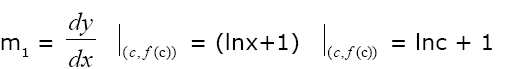slope of the line joining (1, 0), (e, e)

m2 = (e/e-1)

m2 = m1 ⇒ ln c+1 = e/(e-1)

ln c = (e/e-1)-1

= 1/(e-1)

c = e(1/e-1)

7. If y = ((2/π)x-1) cosec x is the solution of the differential equation, (dy/dx)+p(x)y = ((2/π)-1)cosec x, 0<x,π/2, then the function p(x) is equal to:

1) cosec x
2) cot x
3) tan x
4) sec x

y = ((2/π)x-1)cosec x

Differentiate w.r.t.x

(dy/dx) = (2/π)cosec x-((2x/π)-1)cosec x.cot x

(dy/dx) +(2x/π-1)cosec x.cot x = (2/π) cosec x

(dy/dx)+y cot x = (2/π) cosec x

Compare this differential equation with given differential equation

p(x) = cot x

8. If α and β are the roots of the equation 2x(2x+1) = 1, then β is equal to:

1) 2α(α-1)
2) -2α(α+1)
3) 2α2
4) 2α(α+1)

2x(2x+1) = 1

If α and β are the roots i.e α and β satisfy this equation

2α(2α+1) = 1

⇒ α(2α+1) = ½

4x2+2x-1 = 0

α + β = -1/2

= -α(2α+1)

β = -α(2α+1)-α

= -α(2α+2)

= -2α(α+1)

9. For all twice differentiable functions f: R→ R, with f(0) = f(1) = f’(0) = 0,

1) f”(x) = 0, at every point x∈(0,1)
2) f”(x) ≠ 0, at every point x∈(0,1)
3) f”(x) = 0, for some x∈(0,1)
4) f”(0) = 0

Applying rolle’s theorem in [0,1] for function f(x)

f’(c) = 0, c ∈(0,1)

again applying rolles theorem in [0,c] for function f'(x) s

f’’(c1) = 0, c1∈(0,c)

10. The area (in sq.units) of the region enclosed by the curves y = x2-1 and y = 1-x2 is equal to:

1) 4/3
2) 7/2
3) 16/3
4) 8/3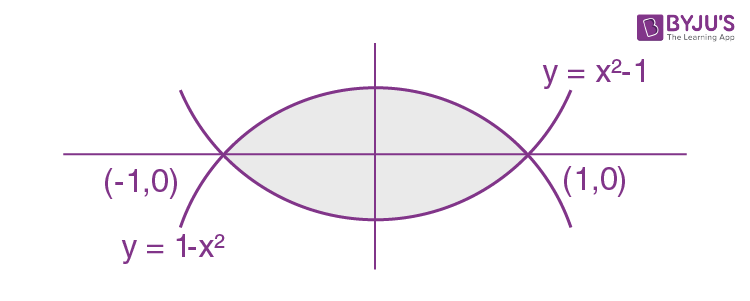Total area =

$$\begin{array}{l}4\int_{0}^{1}(1-x^{2})dx = 4[x-\frac{x^{3}}{3}]_{0}^{1}\end{array}$$

= 4(1-1/3)

= 8/3 sq. unit

11. For a suitably chosen real constant a, let a function, f:R-{-a}→R be defined by f(x) = (a-x)/(a+x). further suppose that for any real number x ≠ -a and f(x) ≠ -a, (fof)(x) = x. Then f(-1/2) is equal to:

1) -3
2) 3
3) 1/3
4) -1/3

f(x) = (a-x)/(a+x)

f(f(x)) = (a-f(x))/(a+f(x)) = x

(a-ax)/(1+x) = f(x) = (a-x)/(a+x)

a(1-x)/(1+x) = (a-x)/(a+x)

⇒ a = 1

So f(x) = (1-x)/(1+x)

f(-1/2) = 3

12. Let θ = π/5 and A =

$$\begin{array}{l}\begin{bmatrix} \cos \theta & \sin \theta \\ – \sin \theta & \cos \theta \end{bmatrix}\end{array}$$
. If B = A+A4, then det (B):

1) is one
2) lies in (1,2)
3) lies in (2,3)
4) is zero

$$\begin{array}{l}\begin{bmatrix} \cos \theta & \sin \theta \\ – \sin \theta & \cos \theta \end{bmatrix}\end{array}$$

B = A+A4

A2 =

$$\begin{array}{l}\begin{bmatrix} \cos \theta & \sin \theta \\ – \sin \theta & \cos \theta \end{bmatrix}\begin{bmatrix} \cos \theta & \sin \theta \\ – \sin \theta & \cos \theta \end{bmatrix}\end{array}$$

=

$$\begin{array}{l}\begin{bmatrix} \cos 2\theta & \sin 2\theta \\ – \sin 2\theta & \cos 2\theta \end{bmatrix}\end{array}$$

Similarly

A4 =

$$\begin{array}{l}\begin{bmatrix} \cos 4\theta & \sin 4\theta \\ – \sin 4\theta & \cos 4\theta \end{bmatrix}\end{array}$$

B = A4+A

=

$$\begin{array}{l}\begin{bmatrix} \cos 4\theta & \sin 4\theta \\ – \sin 4\theta & \cos 4\theta \end{bmatrix}+\begin{bmatrix} \cos \theta & \sin \theta \\ – \sin \theta & \cos \theta \end{bmatrix}\end{array}$$

=

$$\begin{array}{l}\begin{bmatrix} \cos 4\theta +\cos \theta & \sin 4\theta+\sin \theta \\ – \sin 4\theta-\sin \theta & \cos 4\theta +\cos \theta \end{bmatrix}\end{array}$$

det B = (cos4θ+cos θ)2 +(sin 4θ+sin θ)2

= cos24θ + cos2θ+2cos 4θ cos θ+sin24 θ + sin2 θ+2sin4 θ sin θ

= 2+2(cos4θ cos θ+sin4 θ sin θ)

= 2+2 cos3 θ

At θ = π/5,

det B = 2+2 cos3×π/5

= 2(1-sin 18)

= 2(1-(√5-1)/4)

= 2(5-√5)/4)

= (5-√5)/2

lies in (1,2)

13. The centre of the circle passing through the point (0,1) and touching the parabola y = x2 at the point (2,4) is :

1) (3/10, 16/5)
2) (6/5, 53/10)
3) (-16/5, 53/10)
4) (-53/10, 16/5)

Circle passing through point (0,1) and touching curve y = x2 at (2,4)

tangent at (2,4) is (y+4)/2 = x(2)

⇒ y-4x+4 = 0

Equation of circle

(x-2)2 +(y-4)2+λ(4x-y-4) = 0

Passing through (0,1) 4+9 +λ(-5) = 0

λ = 13/5

Circle is

x2-4x+4+y2-8y+16+(13/5)(4x-y-4) = 0

x2+y2+((52/5)-4)x-(8+13/5)y+20-(52/5) = 0

x2+y2+(32/5)x-(53/5)y+(48/5) = 0

centre is (-16/5, 53/10)

14. A plane P meets the coordinate axes at A, B and C respectively. The centroid of a triangle ABC is given to be (1,1,2). Then the equation of the line through this centroid and perpendicular to the plane P is:

1) (x-1)/2 = (y-1)/1 = (z-2)/1
2) (x-1)/2 = (y-1)/2 = (z-2)/1
3) (x-1)/1 = (y-1)/2 = (z-2)/2
4) (x-1)/1 = (y-1)/1 = (z-2)/2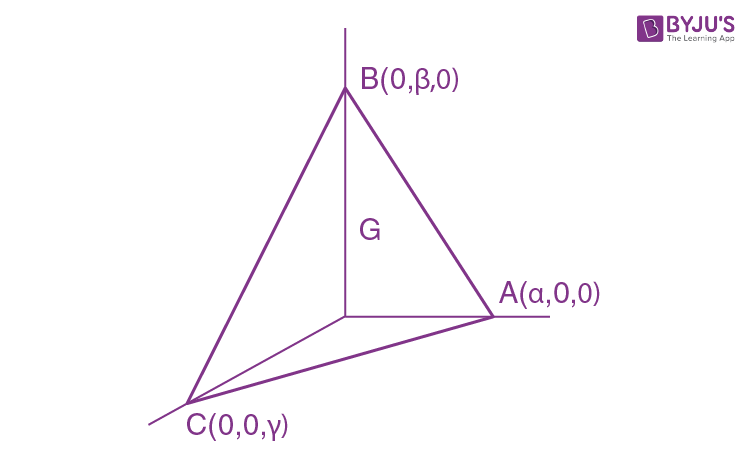G = (α/3, β/3,

$$\begin{array}{l}\gamma\end{array}$$
/3) = (1, 1, 2)

α = 3, β = 3,

$$\begin{array}{l}\gamma\end{array}$$
= 6

Equation of plane is

(x/α)+(y/β)+(z/

$$\begin{array}{l}\gamma\end{array}$$
) = 1

(x/3)+(y/3)+(z/6) = 1

2x+2y+z = 6

Required line perpendicular to plane is (x-1)/2 = (y-1)/2 = (z-2)/1

15. Let f : R→ R be a function defined by f(x) = max {x,x2}. Let S denote the set of all points in R, where f is not differentiable. Then

1) {0,1}
2) an empty set
3) {1}
4) {0}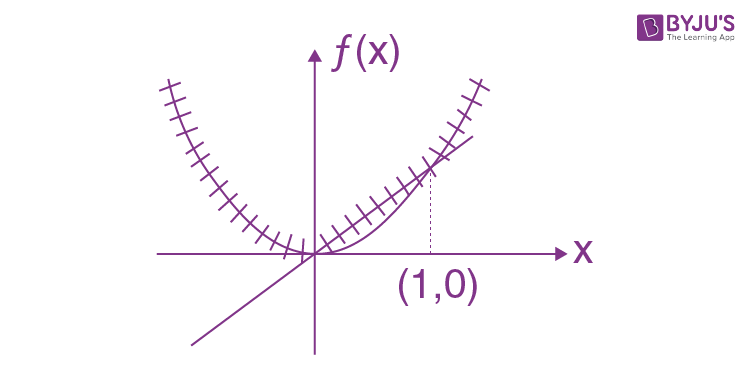Function is not differentiable at two point {0,1}.

16. The angle of elevation of the summit of a mountain from a point on the ground is 450. After climbing up one km towards the summit at an inclination of 300 from the ground, the angle of elevation of the summit is found to be 600. Then the height (in km) of the summit from the ground is: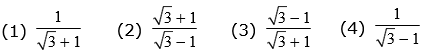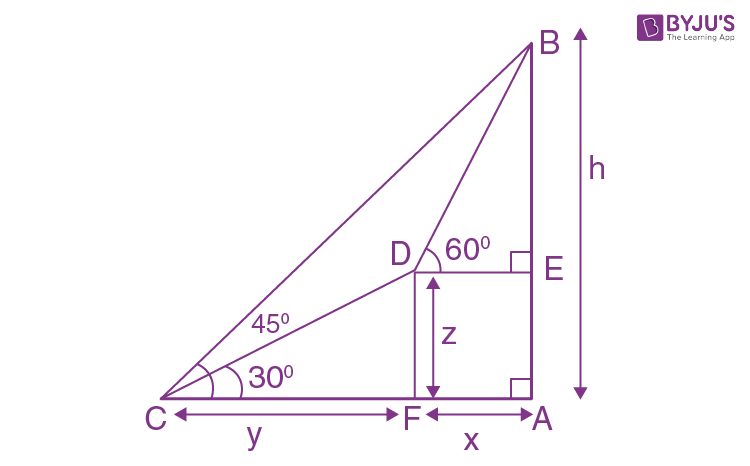If ΔCDF

Sin 300 = z/1

Z = ½ Km

Cos 300 = y/1

y = √3/2 Km

Now in ABC

tan 450 = h/(x+y)

⇒ h = x+y

Or x = h -√3/2

Now, in ΔBDE

tan 600 = (h-z)/x

After substituting the value of x, we get

h = 1/ (√3-1) Km

17. If the constant term in the binomial expansion of (√x – k/x2)10 is 405, then |k| equals:

1) 1
2) 9
3) 2
4) 3

10Cr (-k/x2)r (√x)10-r

10Cr (-k)r (x)5-5r/2

For constant term

5-5r/2 = 0

Or r = 2

Now, T3 = 10C2 k2 = 405

Or k2 = 405/45 = 9

Or |k| = 3

18. Let z = x+iy be a non-zero complex number such that z2 = i|z|2, where i = √-1 , then z lies on the

1) line, y = x
2) real axis
3) imaginary axis
4) line, y = -x

z2 = i |z|2

x2-y2+2i xy = i(x2+y2)

equating real terms

x2-y2 = 0

⇒ x2 = y2

equating imaginary terms

2xy = x2+y2

(x-y)2 = 0

⇒ x = y

19. Let L denote the line in the xy-plane with x and y intercepts as 3 and 1 respectively. Then the image of the point (-1, -4) in this line is:

1) (11/5, 28/5)
2) (8/5, 29/5)
3) (29/5, 11/5)
4) (29/5, 8/5)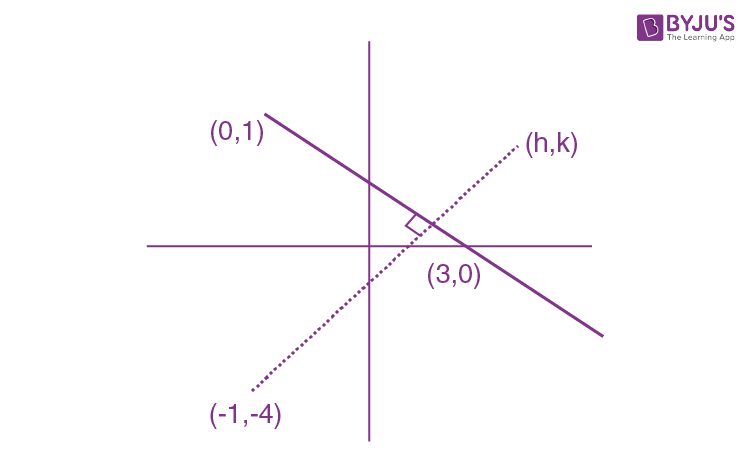Let (h, k) is the image of the point (-1, -4) in the line

x/3 + y/1 = 1

x+3y = 3

L2: 3x-y + λ = 0

-3+4+λ = 0

λ = -1

3x-y = 1

(h,k) satisfy the equation of line L2

3h-k = 1 …(1)

$$\begin{array}{l}|\frac{-1-12-3}{\sqrt{1+9}}| = |\frac{h+3k-3}{\sqrt{1+9}}|\end{array}$$

16 = |h+3k-3|

h +3k = 19 …(2)

h +3k = -13 …(3)

From equation (2) and (3), put value of h in (1)

h = 19-3k,

3(19 -3k) -k=1

-10k = -56 = 28/5

k = 28/5

h = 19-3(28/5) = 11/5

h = -13-3k

3(-13-3k) -k=1

-10k = 40

k = -4

Image = (11/5, 28/5)

20. Consider the statement : “For an integer n, if n3-1 is even, then n is odd.” The contrapositive statement of this statement is:

1) For an integer n, if n is even, then n3-1 is even
2) For an integer n, if n is odd, then n3-1 is even
3) For an integer n, if n3-1 is not even, then n is not odd.
4) For an integer n, if n is even, then n3-1 is odd

P:n3-1 is even, q : n is odd

Contrapositive of p →q = ∼q → ∼p

⇒ If n is not odd then n3-1 is not even

⇒ For an integer n, if n is even, then n3-1 is odd

21. The number of words (with or without meaning) that can be formed from all the letters of the word “LETTER” in which vowels never come together is:

Consonants → LTTR

Vowels → EE

Total No of words = 6!/(2!2!)

= 180 (T and E repeated)

Total no of words if vowels are together

= 5!/2!

= 60 (E is repeated)

Required = 180-60 = 120

22. If

$$\begin{array}{l}\vec{x}\end{array}$$
and
$$\begin{array}{l}\vec{y}\end{array}$$
be two non-zero vectors such that
$$\begin{array}{l}\left | \vec{x}+\vec{y} \right |= \left | \vec{x} \right |\end{array}$$
and
$$\begin{array}{l}2\vec{x}+\lambda \vec{y}\end{array}$$
is perpendicular to
$$\begin{array}{l}\vec{y}\end{array}$$
, then the value of λ is

$$\begin{array}{l}\left | \vec{x}+\vec{y} \right |^{2}= \left | \vec{x} \right |^{2}\end{array}$$

$$\begin{array}{l}\left | \bar{y} \right |^{2}+2\bar{x}.\bar{y}=0\end{array}$$
..(i)

And

$$\begin{array}{l}(2\bar{x}+\lambda \bar{y}).\bar{y}= 0\end{array}$$

$$\begin{array}{l}\lambda (\left | \bar{y} \right |)^{2}+2\bar{x}\bar{y}=0\end{array}$$
..(ii)

by comparing (i) and (ii)

we get λ = 1

23. Consider the data on x taking the values 0, 2, 4, 8, …..,2n with frequencies nC0, nC1, nC2… nCn, respectively. If the mean of this data is 728/2n, then n is equal to:

 Xi 0 2 22 … 2n fi nC0 nC1 nC2 … nCn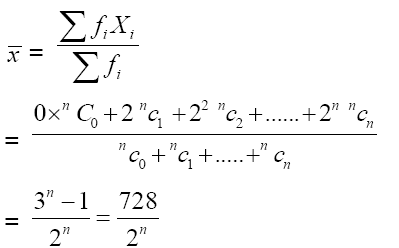3n = 729 = 36

n = 6

24. Suppose that function f : R→R satisfies f(x+y) = f(x)f(y) for all x, y∈R and f(1) = 3. If

$$\begin{array}{l}\sum_{i=1}^{n}f(i)=363\end{array}$$
, then n is equal to:

f(x+y) = f(x) f(y)

f(x) = ax

f(1) = a = 3

So f(x) = 3x

$$\begin{array}{l}\sum_{i=1}^{n}f(i)=363\end{array}$$

⇒ 3+32+33+….+3n = 363

⇒ 3(3n-1)/2 = 363

⇒ n = 5

25. The sum of distinct values of λ for which the system of equations

(λ-1)x+(3λ+1)y+2λz = 0

(λ-1)x+(4λ-2)y+(λ+3)z = 0

2x+(3λ+1)y+3(λ-1)z = 0 has non-zero solutions, is:

$$\begin{array}{l}\begin{vmatrix} \lambda -1 & 3\lambda +1 & 2\lambda \\ \lambda -1 & 4\lambda -2 &\lambda +3 \\ 2 & 3\lambda +1 & 3(\lambda -1) \end{vmatrix}=0\end{array}$$

R2 → R2-R1

R3 → R3-R1

$$\begin{array}{l}\begin{vmatrix} \lambda -1 & 3\lambda +1 & 2\lambda \\ 0 & \lambda -3 &-\lambda +3 \\ 3-\lambda & 0 & (\lambda -3) \end{vmatrix}=0\end{array}$$

C1 → C1 + C3

$$\begin{array}{l}\begin{vmatrix} 3\lambda -1 & 3\lambda +1 & 2\lambda \\ -\lambda +3& \lambda -3 &-\lambda +3 \\ 0 & 0 & (\lambda -3) \end{vmatrix}=0\end{array}$$

(λ-3)[(3λ-1)(λ-3)-3(3-λ)(3λ+1)] = 0

(λ-3)[3λ2-10λ+3-(8λ-3λ2+3)] = 0

(λ-3)(6λ2-18λ) = 0

(6λ)(λ-3)2 = 0

λ = 0, 3

Sum of the values of λ = 0+3 = 3